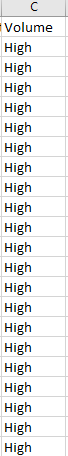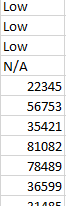# IF Condition Requird

I am Having Data Like below Image.
If it is below 2 Cr or N/A should be write “LOW” in same column
If it is above 2Cr should write “High”
Writing “High” For all Cell’sWhy do you want to write the data in the same column? any specific reason?

Regards
Sudharsan

It is Just Task that i need to write.

Can you share the Sample file here? @Manju_Reddy_Kanughula

HistoricalData_1677305614628.xlsx (11.3 KB)

Instead of using Write cell use the Assign Activity @Manju_Reddy_Kanughula

Use the conditions seperately
Main if Condition

``````CurrentRow("Volume").ToString.Trim.Contains("N/A")
``````

In Then
Left Side → CurrentRow(“Volume”)
Right Side → “Low”

In Else

Else If Activity and first condition

``````CInt(CurrentRow("Volume").ToString)<= 20000000
``````

Left Side → CurrentRow(“Volume”)
Right Side → “Low”

Else If Second Condition

``````CInt(CurrentRow("Volume").ToString)> 20000000
``````

Left Side → CurrentRow(“Volume”)
Right Side → “High”

Out side/Below the For each row

Use write range Activity and then write the same datatable whih you used in the For eacg row In Datatable activity

Regards
Sudharsan

Try this code inside an invoke code activity and pass the datatable in the arguments.

Dt.AsEnumerable.ToList.ForEach(Sub (row) row(“Column_Name_toWriteRemark”) = If((Convert.ToDouble(row(“Volume”))<=20000000 or Convert.ToDouble(row(“Volume”)) = 0),“High”,“Low”))

instead of “Column_Name_toWriteRemark” pass your column name in which you need to write the data as high or low.